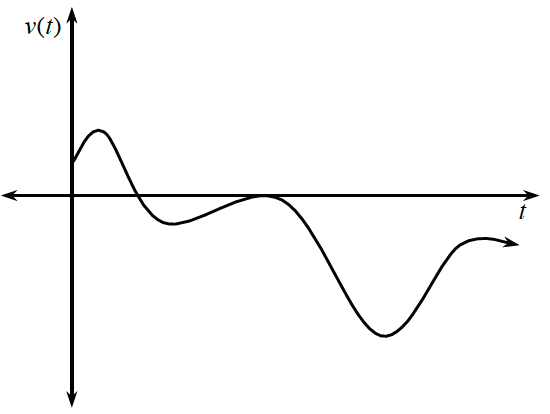### Home > CALC3RD > Chapter Ch1 > Lesson 1.4.4 > Problem1-181

1-181.The graph at right represents the velocity of an object as a function of time. Trace it on your paper. Homework Help ✎

1. Put a star (*) at the point where velocity is the greatest.

What is the maximum point on the graph?

2. With another color, sketch a graph of the speed on the same set of axes.

Speed is the absolute value of velocity. Flip all the negative y-values to their corresponding positive positions.

3. Indicate with a double star (**) the position where speed is the greatest.

What is the maximum point on the speed graph? Notice that this is NOT the same as the maximum point on the velocity graph.

4. Explain why the greatest velocity and the greatest speed do not occur at the same position.

The maximum is the greatest positive value. How does changing the graph to an absolute value graph change the possible maximum?

5. Sketch a new graph where speed and velocity have the same maximum value.

A correct velocity graph will have a maximum above the x-axis and a minimum below the x-axis, but the maximum point is further from the x-axis than the minimum point. In other words, |maximum| > |minimum|.